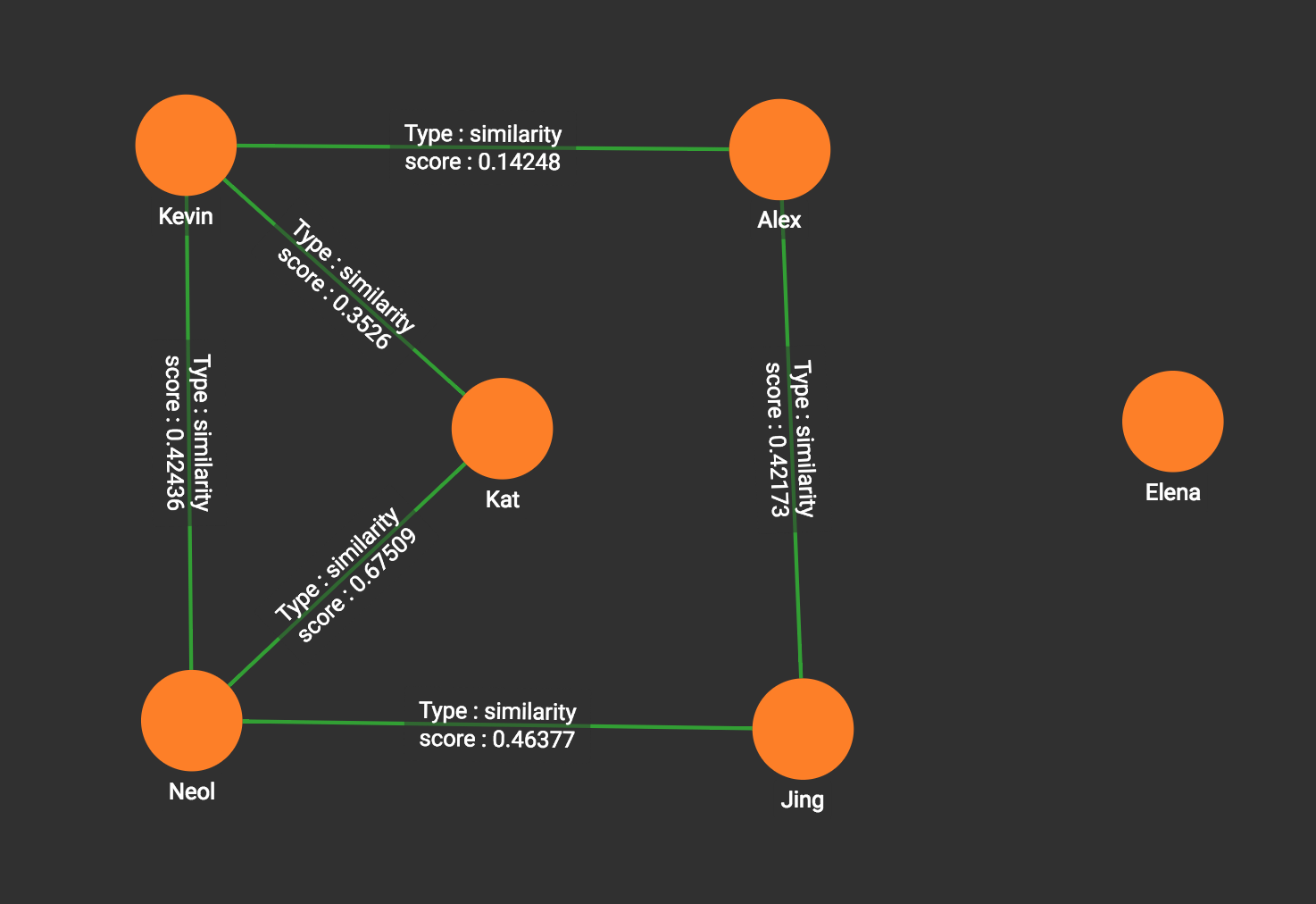# Cosine Similarity of Neighborhoods (All Pairs)

This algorithm computes the same similarity scores as the cosine similarity of neighborhoods, single-source algorithm (`cosine_nbor_ss`), except that it considers ALL pairs of vertices in the graph (for the vertex and edge types selected by the user). Naturally, this algorithm will take longer to run. For very large and very dense graphs, this may not be a practical choice.

## Specifications

``````tg_cosine_nbor_ap (SET<STRING> v_type, SET<STRING> e_type, SET<STRING> re_type,
STRING weight, INT top_k, INT output_limit, BOOL print_accum = TRUE,
STRING similarity_edge = "", STRING file_path = "")``````
Characteristic Value

Result

The top k vertex pairs in the graph which have the highest similarity scores, along with their scores.

The result is available in three forms:

• streamed out in JSON format

• written to a file in tabular format, or

• stored as a vertex attribute value.

Input Parameters

• `SET<STRING> v_type`: Vertex types to calculate cosine similarity score for

• `SET<STRING> e_type`: Edge types to traverse

• `SET<STRING> re_type`: Reverse edge types to traverse

• `STRING weight`: Edge attribute to use as weight

• `INT top_k`: the number of vertex pairs with the highest similarity scores to return

• `INT output_limit`: If >=0, max number of vertices to output to JSON.

• `BOOL print_accum`: If `true, output JSON to standard output.`

• `STRING similarity_edge`: If provided, the similarity score will be saved to this edge

• `filepath`(for file output only): the path to the output file

Result Size

`top_k`

Time Complexity

O(E), E = number of edges

Graph Types

Undirected or directed edges, weighted edges

## Example

Using the movie graph, calculate the cosine similarity between all pairs and show the top 5 pairs. This is the JSON result:

``````[
{
"@@total_result": [
{
"vertex1": "Kat",
"vertex2": "Neil",
"score": 0.67509
},
{
"vertex1": "Jing",
"vertex2": "Neil",
"score": 0.46377
},
{
"vertex1": "Kevin",
"vertex2": "Neil",
"score": 0.42436
},
{
"vertex1": "Jing",
"vertex2": "Alex",
"score": 0.42173
},
{
"vertex1": "Kat",
"vertex2": "Kevin",
"score": 0.3526
}
]
}
]``````

The FILE output is similar to the output of cosine_nbor_file.

The ATTR version will create k edges: